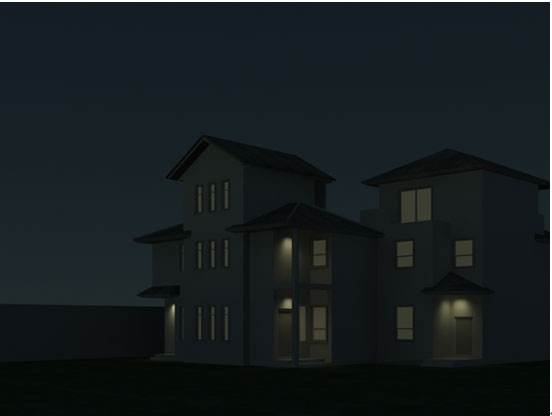2019-02-17 21:15:44 qq_34725005 阅读数 211
• ###### 学习OpenCV3.2+QT5+ffmpeg实战开发视频编辑器视频教程

OpenCV3.2+QT5+ffmpeg实战开发视频编辑器视频培训课程概况：教程中会讲解到基于opencv视频和摄像机录制、播放和播放进度控制，多视频图像合并、多视频图像融合、剪切、视频亮度、对比度、尺寸（近邻插值（手动实现），双线性插值，图像金字塔）、颜色格式（灰度图，二值化（阈值）），旋转镜像，视频裁剪（ROI），视频水印（ROI+weight），导出处理后的视频（包含音频，使用ffmpeg工具对音频进行抽取、剪切和终于opencv处理的视频合并）。

19573 人正在学习 去看看 夏曹俊
``````import cv2
import numpy as np

def convert(r):
s = np.empty(r.shape,dtype=np.uint8)
for j in range(r.shape):
for i in range(r.shape):
s[j][i]=(r[j][i]/255)**0.67*255
return s

im_mat = np.asarray(im)
im_converted_mat = convert(im_mat)
cv2.imwrite('tetet2.jpg',im_converted_mat)
cv2.imshow('original',im)
cv2.imshow('img',im_converted_mat)
cv2.waitKey()
``````2019-07-17 15:38:42 space_walk 阅读数 281
• ###### 学习OpenCV3.2+QT5+ffmpeg实战开发视频编辑器视频教程

OpenCV3.2+QT5+ffmpeg实战开发视频编辑器视频培训课程概况：教程中会讲解到基于opencv视频和摄像机录制、播放和播放进度控制，多视频图像合并、多视频图像融合、剪切、视频亮度、对比度、尺寸（近邻插值（手动实现），双线性插值，图像金字塔）、颜色格式（灰度图，二值化（阈值）），旋转镜像，视频裁剪（ROI），视频水印（ROI+weight），导出处理后的视频（包含音频，使用ffmpeg工具对音频进行抽取、剪切和终于opencv处理的视频合并）。

19573 人正在学习 去看看 夏曹俊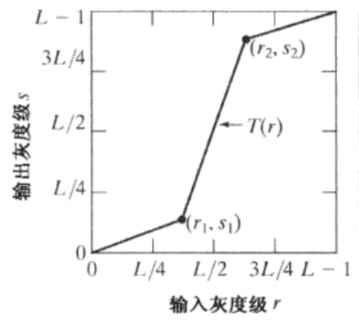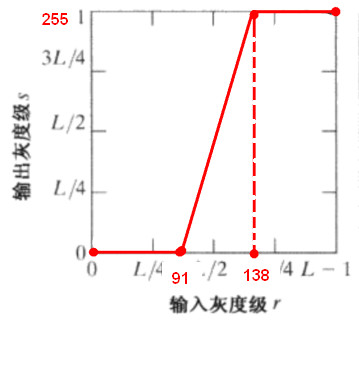``````% 读入图像

% max()：返回一个行向量，向量的第i个元素是矩阵A的第i列上的最大值。
% 再一次即可求得最高灰度级
r_max = max(max(img_1));
% 与 max() 同理
r_min = min(min(img_1));

% 求输入图像的尺寸
[r, c] = size(img_1);

% 生成两张0图，一张用于对比度拉伸，一张用于阈值处理
img_2 = zeros(r, c);
img_3 = zeros(r, c);

% 将输入图像转换成double型
img_1s = double(img_1);

% 计算斜率
k = (255 - 0) / (r_max - r_min);
% 灰度级总和
sum = 0;

for x = 1 : r
for y = 1 : c
% 对每个像素点进行拉伸，并赋值到新图像
img_2(x, y) = k * (img_1s(x, y) - r_min);
% 求灰度级总和
sum = sum + img_1s(x, y);
end
end

% 求阈值，这里阈值为平均值
avg = sum / (r * c);

% 阈值处理
for x = 1 : r
for y = 1 : c
% 小于阈值赋值0
if img_1s(x, y) < avg
img_3(x, y) = 0;
% 大于阈值赋值1
else
img_3(x, y) = 1;
end
end
end

% 转换
img_2 =  im2uint8(mat2gray(img_2));
img_3 =  im2uint8(mat2gray(img_3));
% 显示
subplot(131), imshow(img_1);
subplot(132), imshow(img_2);
subplot(133), imshow(img_3);
``````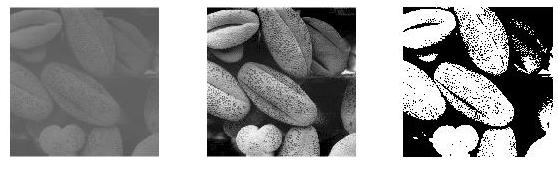2020-01-06 22:10:26 qiu931110 阅读数 20
• ###### 学习OpenCV3.2+QT5+ffmpeg实战开发视频编辑器视频教程

OpenCV3.2+QT5+ffmpeg实战开发视频编辑器视频培训课程概况：教程中会讲解到基于opencv视频和摄像机录制、播放和播放进度控制，多视频图像合并、多视频图像融合、剪切、视频亮度、对比度、尺寸（近邻插值（手动实现），双线性插值，图像金字塔）、颜色格式（灰度图，二值化（阈值）），旋转镜像，视频裁剪（ROI），视频水印（ROI+weight），导出处理后的视频（包含音频，使用ffmpeg工具对音频进行抽取、剪切和终于opencv处理的视频合并）。

19573 人正在学习 去看看 夏曹俊

##### 1.整体实现代码
``````from PIL import Image
from PIL import ImageEnhance
import matplotlib.pyplot as plt
import numpy as np

def get_piexl_list(img):
w, h = img.size, img.size
r_list = []
g_list = []
b_list = []
for i_w in range(w):
for j_h in range(h):
r, g, b = img_list[i_w, j_h]
r_list.append(r)
g_list.append(g)
b_list.append(b)

r_list = np.asarray(r_list)
g_list = np.asarray(g_list)
b_list = np.asarray(b_list)
return r_list,g_list,b_list

def draw_mulit_pic(r_list,g_list,b_list):
fig, ax = plt.subplots()

ax.hist(r_list, bins=256, normed=True, color='yellow')
ax2.hist(g_list, bins=256, normed=True, color='red')
ax3.hist(b_list, bins=256, normed=True, color='blue')

plt.show()

def enhance_contrast(img):
enh_con = ImageEnhance.Contrast(img)
contrast = 1.5
img_contrasted = enh_con.enhance(contrast)
img_contrasted.show()
return img_contrasted

if __name__ == "__main__":
img = Image.open('D:\\1.jpeg')

# 原始图像直方图
r_list,g_list,b_list = get_piexl_list(img)
draw_mulit_pic(r_list, g_list, b_list)

# 对比度增强
img_contrasted = enhance_contrast(img)

# 对比度增强后图像直方图
r_list,g_list,b_list = get_piexl_list(img_contrasted)
draw_mulit_pic(r_list, g_list, b_list)

``````
##### 2.原始图像直方图
``````    img = Image.open('D:\\1.jpeg')

# 原始图像直方图
r_list,g_list,b_list = get_piexl_list(img)
draw_mulit_pic(r_list, g_list, b_list)
``````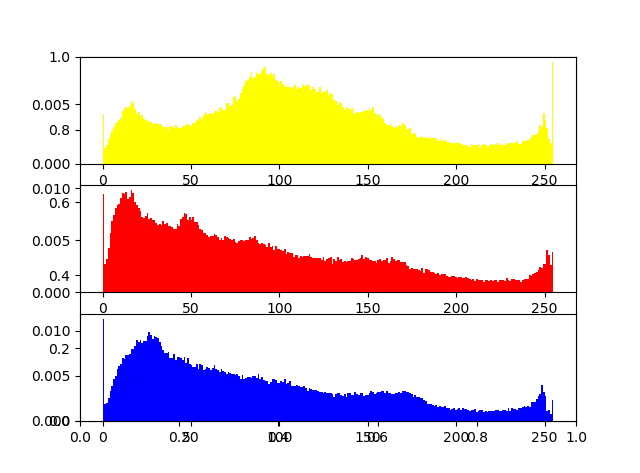##### 3.增强后图像直方图
``````    # 对比度增强
img_contrasted = enhance_contrast(img)

# 对比度增强后图像直方图
r_list,g_list,b_list = get_piexl_list(img_contrasted)
draw_mulit_pic(r_list, g_list, b_list)
``````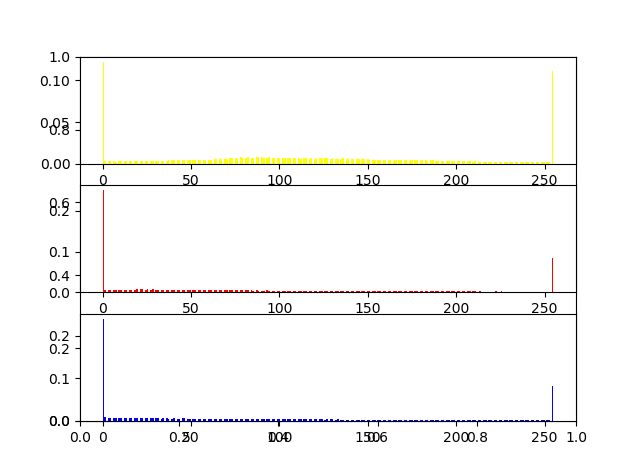##### 4.对比度增强结果显示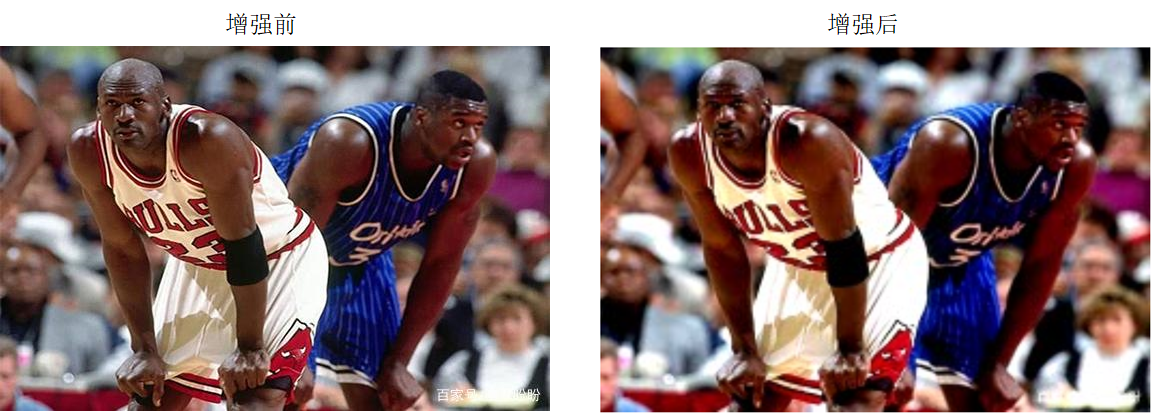2011-11-23 15:31:18 honesty2008 阅读数 8876
• ###### 学习OpenCV3.2+QT5+ffmpeg实战开发视频编辑器视频教程

OpenCV3.2+QT5+ffmpeg实战开发视频编辑器视频培训课程概况：教程中会讲解到基于opencv视频和摄像机录制、播放和播放进度控制，多视频图像合并、多视频图像融合、剪切、视频亮度、对比度、尺寸（近邻插值（手动实现），双线性插值，图像金字塔）、颜色格式（灰度图，二值化（阈值）），旋转镜像，视频裁剪（ROI），视频水印（ROI+weight），导出处理后的视频（包含音频，使用ffmpeg工具对音频进行抽取、剪切和终于opencv处理的视频合并）。

19573 人正在学习 去看看 夏曹俊```function lab1(addr,x1,y1,x2,y2)
%功能：完成实验一，灰度拉伸处理
% x1,y1,x2,y2 为拉伸处理的线段端点坐标，当参数小于5个时
% 这些坐标均采用默认值，可以直接按 F5 运行。
if nargin<1
end
if nargin<5
x1=28;y1=28;
x2=75;y2=255;
end
if  x1==x2
display('x1、x2不能相同，退出程序');
return ;
end
[r,c,n]=size(I);
k=(y1-y2)/(x1-x2);
b=y1-k*x1;
if n>1
I2=rgb2gray(I);
else I2=I;
end
I2=double(I2);
for x=1:r
for y=1:c
tmp=I2(x,y);
if tmp>=x1 && tmp<=x2
I2(x,y)=k*tmp+b;
end
end
end
subplot(1,2,1);imshow(I);title('original image ');
subplot(1,2,2);imshow(I2,[]);title('image after modification');
```

2019-07-14 22:19:44 wujuxKkoolerter 阅读数 156
• ###### 学习OpenCV3.2+QT5+ffmpeg实战开发视频编辑器视频教程

OpenCV3.2+QT5+ffmpeg实战开发视频编辑器视频培训课程概况：教程中会讲解到基于opencv视频和摄像机录制、播放和播放进度控制，多视频图像合并、多视频图像融合、剪切、视频亮度、对比度、尺寸（近邻插值（手动实现），双线性插值，图像金字塔）、颜色格式（灰度图，二值化（阈值）），旋转镜像，视频裁剪（ROI），视频水印（ROI+weight），导出处理后的视频（包含音频，使用ffmpeg工具对音频进行抽取、剪切和终于opencv处理的视频合并）。

19573 人正在学习 去看看 夏曹俊

# 判断图像是否为低对比度图像

Python实现代码如下：

``````def is_low_contrast(src,fraction_threshold=0.5, lower_percentile=1,
upper_percentile=99):
# 转换为灰度图像
src = np.asanyarray(src)
if src.ndim == 3 and src.shape in [3, 4]:
src = cv2.cvtColor(src,cv2.COLOR_RGB2GRAY)
# 获取图像数据类型的最大值和最小值
dlimits = (np.iinfo(src.dtype).min, np.iinfo(src.dtype).max)
# 计算低亮度像素在整个图像中的范围
limits = np.percentile(src, [lower_percentile, upper_percentile])
# 计算比例
ratio = (limits - limits) / (dlimits - dlimits)
return ratio < fraction_threshold
``````